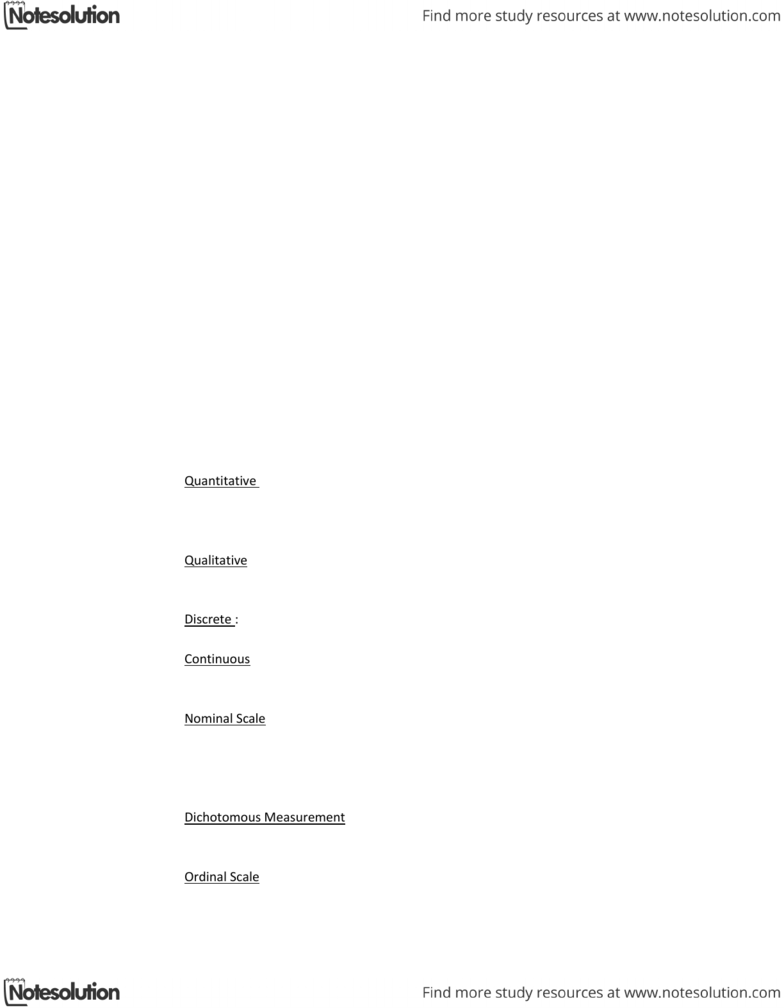Class Notes (1,100,000)
CA (620,000)
UTSG (50,000)
ARH (50)
Lecture 2

# ARH312Y1 Lecture Notes - Lecture 2: Criterion Validity, Epipaleolithic, Measurement Uncertainty

Department
Archaeology
Course Code
ARH312Y1
Professor
Geoffrey Mac Donald
Lecture
2

Page:
of 4ARH312 Week 2- September 20th
Measurement Theory
Data- observations and measurement you make on objects this data is theory laden (not
independent from theory)
Measurement is comparison- either to a standard (mm etc.) or to a concept
There are measurement institutes that have standards for all weights lengths and
concepts etc.
Types of Measurement
Direct Measurement directly compare an object of interest to a standard
Indirect Measurement- measuring one phenomena to derive measurement of another concept
Validity
Degree of correlation between our measurement and the qualities or values we’re trying to
measure
Face validity = widespread agreement by experts in the field
Content validity = appears to contain all important concepts and behaviours
Criterion validity = comparison of the measurement with a standard
Scales of Measurement
What are some ways we can classify measurements?
Quantitative : anything with numbers
Length
Width
Mass
Qualitative : anything without numbers
Colour
Texture
Discrete : no grey areas between the categories
Apples, oranges and tomatoes
Continuous : between any two numbers on the scale you can find other numbers
The difference between 1cm and 2cm there can be 1.5cm there is always
another number between the numbers you are looking at hence continuous
Nominal Scale : Categories
Unordered and equal weight
Enumeration is the count of object we assign to each class in the nominal scale
Often discrete
Examples are male female or the comparison of pottery (you often just count)
Dichotomous Measurement : one of the other
Male or Female (can be argued)
Present or absent
Ordinal Scale : Categories
With order
Chronological groups are ordinal (ex. Epipaleolithic, Neolithic etc.)
No information of the magnitude if difference between categories
Often discrete
Interval Scale: continuous data and zero is an arbitrary number
Quantitative scale of measurement
Ratio Scale : when there is zero nothing exists
Quantitative scale of measurement
Measurement Errors
Tell us about the quality of our data and they assure us the differences seen in the data are real
Discrete data errors
Misclassification
Ambiguous categories
Source of error: perception and senses
Continuous data errors
Any two values have an infinity of other values between them
Source of error: measurement uncertainty (how certain is your ruler)
Outliers : surprising values in your data caused by inter observer error, intra observer error or
contamination
Accuracy : the degree of bias
Precision : the spread of range of the results
Presenting Data Honestly and Realistically
Let’s say you measure the thickness of an artifact as 3.55mm. Is that reasonable?
This is dishonest because you are pretending you know more precisely then you do
This can affect your statistics
Significant Digits
Related to how accurately and precisely you actually measured something
NOT the number of decimal places
Independent of the scale of the units (mm, cm etc.)
3.2cm = 32mm = 0.032m = 0.000032km, in each case exactly TWO significant digits
Precision and Reliability
Using a reliable, high precision measuring instrument allows you consistently to get the same
result within a very small margin
Does this ensure that the result will be accurate?
Measuring instruments can still be biased, which is why we need to calibrate it
Instrument Precision
The precision of your measuring tool
Descriptive Statistics
Numeric summaries of interval or ratio scale data
Often showing measures of central tendencies
Mean= average
Median= divides observations in half
Mode= highest peak
Measuring Dispersion
How is the data distributed?
Range= difference between the highest and lowest value
Interquartile range= range of middle 50%
Variance= how far away are all your measurement from the mean
Square the deviation from the mean and sum them
Produces an average deviation from the mean but in square units
Standard Deviation
Square root of the variance
Average deviation from the mean
Standard Error
The standard deviation of the sampling distribution of a statistic
Accumulating Error
Generally , when you combine measurements the errors get somewhat bigger
If you add together two measurements the error on the sum is the square root of the
sum of the squared errors
Minimizing or Controlling Error
Pilot projects or test runs
Training and manuals
Double-checks (statistical quality control)
Control groups
Multiple measures of the same object
Calibrating the Balance
Hold down the ON button for 5 seconds to get a menu
Wait for CAL and press ON flash C
Wait for flashing 200
Put on 200g weight and press ON
C and then DONE
Calipers
Manual calipers measure within 0.1mm
Measure exteriors on one side and interiors on the other
Read measurement to the nearest mm on the main scale
Then look on the 0.1mm scale to see where the ticks line up
Measuring Grids
Chart length and chart width
Measuring Box
Need to be able to stance a sherd (place sherd on paper rock it back and forth till it sits
somewhat flat no light is coming through this is what it would look like after being stanced and
what the full piece would look like) Stance the sherd on the edge of the box